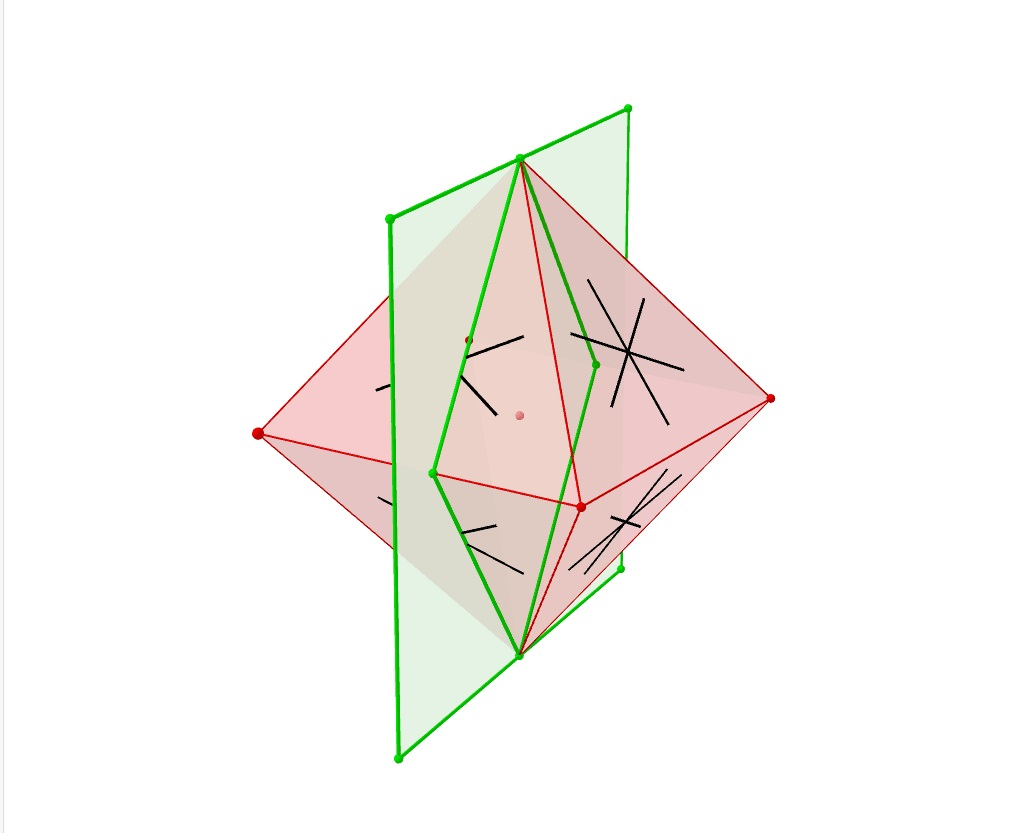# What is the inradius of the octahedron with sidelength a?1) Determine the height of one of twoCarly Shannon 2022-01-21 Answered

What is the inradius of the octahedron with sidelength a?
1) Determine the height of one of two constituent square pyramids by considering a right triangle, using the fact that the height of the equilateral triangle is $a\sqrt{\frac{3}{2}}$. (Incidentally, by this you obtain the circumradius of the Octahedron.)
2) Now cut the (solid) octahedron along 4 of these latter heights, i.e. across two non-adjacent vertices and two midpoints of parallel sides of the ''square base''. (Figure 1)
Consider the resultant rhombic polygon, whose sidelength is $a\sqrt{\frac{3}{2}}$. Choose a convenient pair of adjacent corners A and B, s.t. the angle in A is obtuse. Then the insphere touches AB, say in M.
So the question becomes: Is there a still simpler proof?You can still ask an expert for help

• Questions are typically answered in as fast as 30 minutes

Solve your problem for the price of one coffee

• Math expert for every subject
• Pay only if we can solve itizumrledk
Step 1
An analytic solution: If we center the octahedron on the origin, this problem amounts to finding the sphere centered at the origin that’s tangent to all of the faces. By symmetry, we only need to consider one face. A plane with equation
$Ax+By+Cz+D=0$
is tangent to the sphere
${x}^{2}+{y}^{2}+{z}^{2}={r}^{2}$
iff the coefficients satisfy the dual quadric equation
${A}^{2}+{B}^{2}+{C}^{2}=\frac{{D}^{2}}{{r}^{2}}$
W.l.o.g. we can place the three vertices at and .
The plane through these points has equation
$\left[\begin{array}{cccc}x& y& z& 1\\ \frac{a}{2}& \frac{a}{2}& 0& 1\\ -\frac{a}{2}& \frac{a}{2}& 0& 1\\ 0& 0& \frac{a}{\sqrt{2}}& 1\end{array}\right]=0$
which simplifies to $2y+\sqrt{2z}=a$ Substituting the coefficients of this plane equation into the dual quadric equation produces $\frac{{a}^{2}}{{r}^{2}}=6$, therefore $r=\frac{a}{\sqrt{6}}$
###### Not exactly what you’re looking for?Roman Stevens

Step 1
Consider this full elementar geometrical derivation:
Inscribe into the octahedron its dual cube. Then the searched for inradius of the octahedron would be the circumradius of that cube. Let the edgelength of the octahedron be a and that of the inscribed dual cube be c.
Next consider a variation of that dual cube as to be an accordingly oriented square prism of varying height, still with vertices on the faces of the octahedron. That prism would have base sides of size
$b=\frac{1}{\sqrt{2}}a×t$
where t is some variation parameter, starting with $t=0$ at the top and ending with $t=1$ at the equatorial section of the octahedron. The corresponding height of that prism then is
$h=\sqrt{2}a\left(1-t\right)$
For the prism to become a cube we have $b=h$ or
$\frac{1}{\sqrt{2}}a×t=\sqrt{2}a\left(1-t\right)$
Solving for t results in $t=\frac{2}{3}$ or
$c=b{\mid }_{t=\frac{2}{3}}=\frac{1}{\sqrt{2}}a×\frac{2}{3}=\frac{\sqrt{2}}{3}a$
Now the inradius r of the a-sized octahedron is the circumradius of the c-sized cube and thus clearly$r=\frac{\sqrt{3}}{2}c=\frac{\sqrt{3}2×\frac{\sqrt{2}}{3}a=\frac{1}{\sqrt{6}}}{a}$

###### Not exactly what you’re looking for?RizerMix
Sketch of idea. I tend to think of it as follows. Consider an octahedron with points on the x, y, and z axes, at coordinates $±1$. Then by symmetry, the inradius is the distance from the origin to the point , which is $\sqrt{\frac{1}{3}}$. By similarity, the inradius of an octahedron with side length a is $\frac{a}{\sqrt{6}}$. (Im